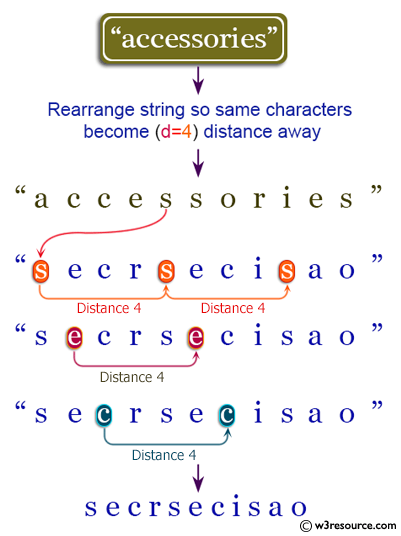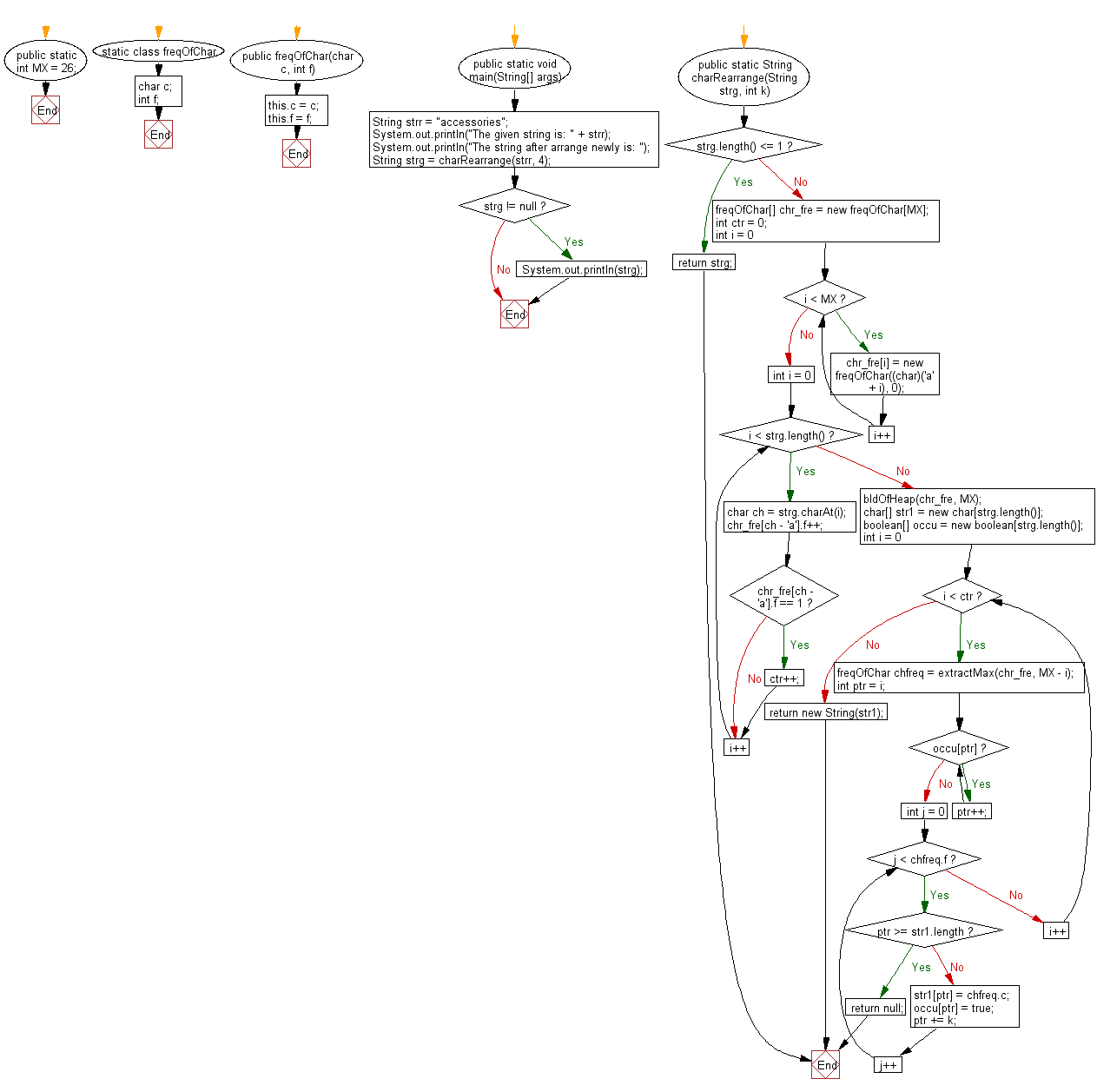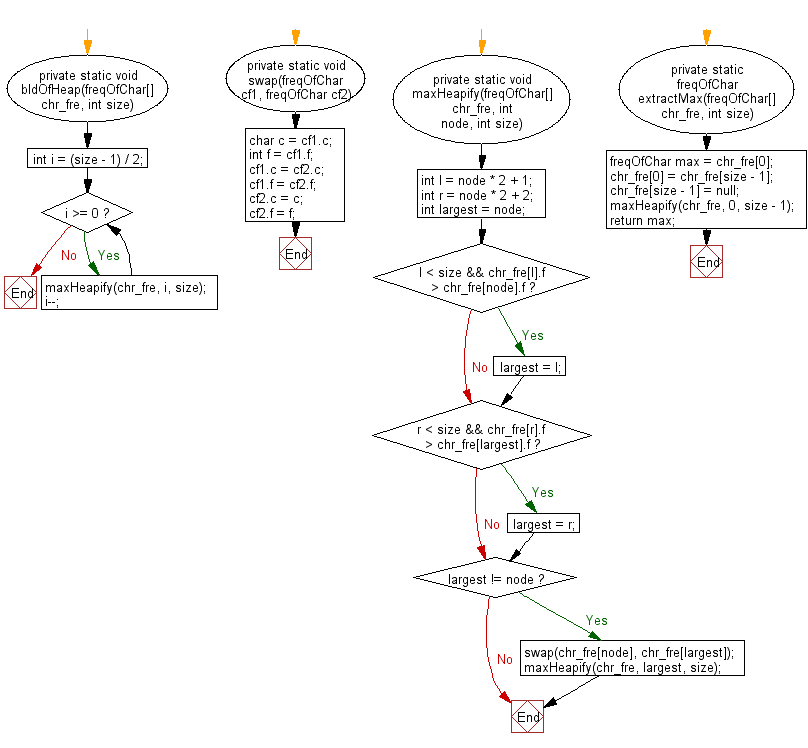﻿ Java exercises: Rearrange a string so that all same characters become d distance away - w3resource# Java String Exercises: Rearrange a string so that all same characters become d distance away

## Java String: Exercise-47 with Solution

Write a Java program to rearrange a string so that all same characters become d distance away.

Pictorial Presentation:Sample Solution:

Java Code:

``````import java.util.*;
public class Main {

public static int MX = 26;
static class freqOfChar {
char c;
int f;
public freqOfChar(char c, int f) {
this.c = c;
this.f = f;
}
}

public static void main(String[] args) {
String strr = "accessories";
System.out.println("The given string is: " + strr);
System.out.println("The string after arrange newly is: ");
String strg = charRearrange(strr, 4);
if (strg != null)
System.out.println(strg);
}

public static String charRearrange(String strg, int k) {
if (strg.length() <= 1) return strg;

freqOfChar[] chr_fre = new freqOfChar[MX];
int ctr = 0;

for (int i = 0; i < MX; i++) {
chr_fre[i] = new freqOfChar((char)('a' + i), 0);
}

for (int i = 0; i < strg.length(); i++) {
char ch = strg.charAt(i);
chr_fre[ch - 'a'].f++;
if (chr_fre[ch - 'a'].f == 1) ctr++;
}

bldOfHeap(chr_fre, MX);

char[] str1 = new char[strg.length()];
boolean[] occu = new boolean[strg.length()];
for (int i = 0; i < ctr; i++) {
freqOfChar chfreq = extractMax(chr_fre, MX - i);
int ptr = i;
while (occu[ptr]) ptr++;

for (int j = 0; j < chfreq.f; j++) {
if (ptr >= str1.length)
return null;
str1[ptr] = chfreq.c;
occu[ptr] = true;
ptr += k;
}
}
return new String(str1);
}

private static void bldOfHeap(freqOfChar[] chr_fre, int size) {
int i = (size - 1) / 2;
while (i >= 0) {
maxHeapify(chr_fre, i, size);
i--;
}
}

private static void swap(freqOfChar cf1, freqOfChar cf2) {
char c = cf1.c;
int f = cf1.f;
cf1.c = cf2.c;
cf1.f = cf2.f;
cf2.c = c;
cf2.f = f;
}

private static void maxHeapify(freqOfChar[] chr_fre, int node, int size) {
int l = node * 2 + 1;
int r = node * 2 + 2;
int largest = node;
if (l < size && chr_fre[l].f > chr_fre[node].f) {
largest = l;
}
if (r < size && chr_fre[r].f > chr_fre[largest].f) {
largest = r;
}
if (largest != node) {
swap(chr_fre[node], chr_fre[largest]);
maxHeapify(chr_fre, largest, size);
}
}
private static freqOfChar extractMax(freqOfChar[] chr_fre, int size) {
freqOfChar max = chr_fre;
chr_fre = chr_fre[size - 1];
chr_fre[size - 1] = null;
maxHeapify(chr_fre, 0, size - 1);
return max;
}
}
```
```

Sample Output:

```The given string is: accessories
The string after arrange newly is:
secrsecisao
```

Flowchart: 1Flowchart: 2Java Code Editor:

Improve this sample solution and post your code through Disqus

What is the difficulty level of this exercise?

﻿

## Java: Tips of the Day

Array vs ArrayLists:

The main difference between these two is that an Array is of fixed size so once you have created an Array you cannot change it but the ArrayList is not of fixed size. You can create instances of ArrayLists without specifying its size. So if you create such instances of an ArrayList without specifying its size Java will create an instance of an ArrayList of default size.

Once an ArrayList is full it re-sizes itself. In fact, an ArrayList is internally supported by an array. So when an ArrayList is resized it will slow down its performance a bit as the contents of the old Array must be copied to a new Array.

At the same time, it's compulsory to specify the size of an Array directly or indirectly while creating it. And also Arrays can store both primitives and objects while ArrayLists only can store objects.

Ref: https://bit.ly/3o8L2KH